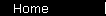### Capital Asset Pricing Model (CAPM)

Equation in modern portfolio theory expressing the idea that securities in the market are priced so that their expected return will compensate investors for their expected risk. The equation is:
r   =   Rf   +   beta x ( Km - Rf )
where
r is the expected return rate on a security;
Rf is the rate of a "risk-free" investment, i.e. cash;
Km is the return rate of the appropriate asset class.
The risk factor beta is calculated in terms of the chosen asset class.

CAPM is used theoretically, to relate securities to the market as a whole, and practically, as the discount rate in discounted cash flow calculations to establish the fair value of an investment.

See the CAPM calculator, and the main article on CAPM.

 home  |  glossary  |  calculator  |  about us  |  books